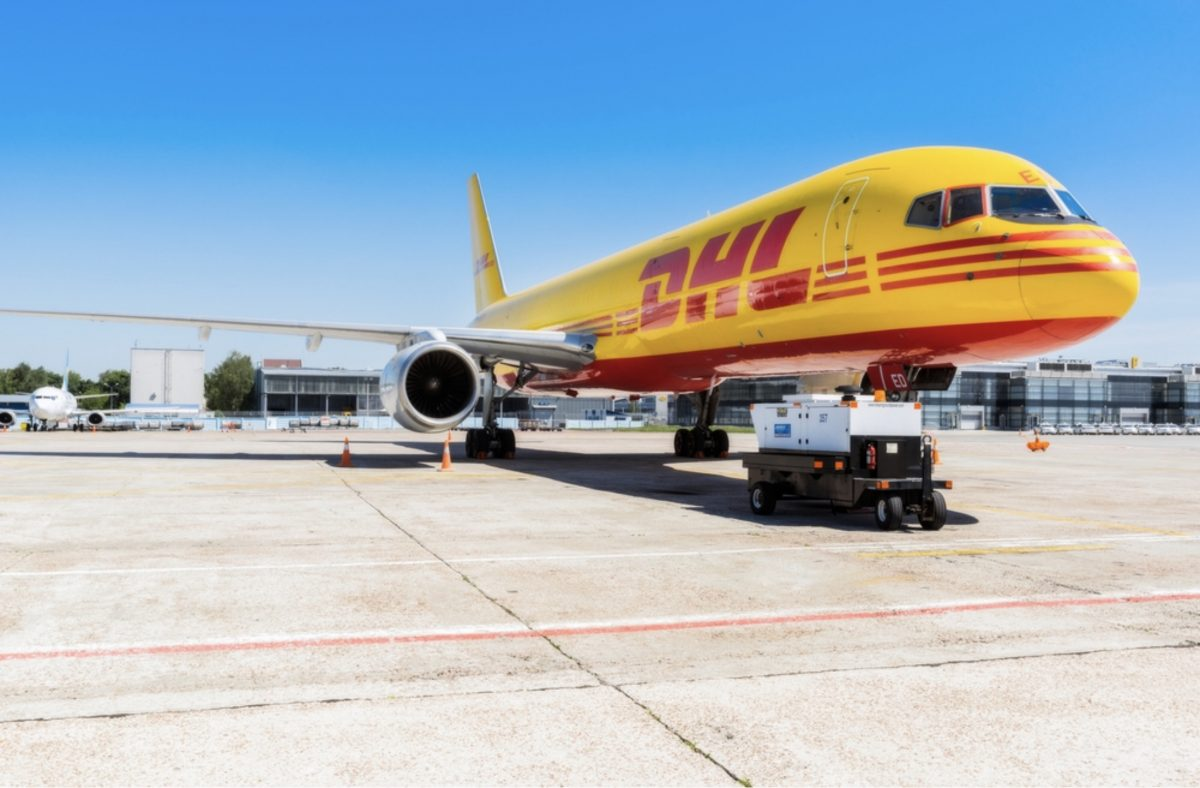Air transportation has an advantage which is faster than sea transportation. Normally, we use Air transportation service for small and light cargo delivery, or use it for urgent delivery case.

Compared with the sea transportation, the air freight is more expensive than the rate of sea shipment.

When you choose the air shipment, you have to understand how much is the air freight for your cargo delivery and you also have to understand how to calculate the air freight.

However, you may think it is difficult for air freight calculation.

If you understand how to calculate air freight, you can compare the benefit of each Air Line services. Also You choose the right decision with the courier service such as DHL and Fedex for light cargo.

Today we would like to explain how to calculate the air freight with actual example.

## How to calculate air freight ?In fact, calculating air freight is not so difficult. We will introduce summary and required element for the calculation of air freight in this chapter.

### The formula of Air freight calculation

if you calculate air freight, you need elements of “Cargo Weight” and “Cargo Volume” and “Rate of Air freight”.

Air freight ＝ Cargo Weight or Cargo volume (Larger One) × Rate of Air Freight

#### Why the Cargo Weight or Volume?

If the cargo volume is large, it takes big space of air plane. In this case, even if the weight is light, the space of air plain will be limited because of this kind of large cargo.

Therefore Air carrier chooses the larger unit of Cargo Weight or Cargo Volume for freight calculation.

We call it as “Chargeable Weight“ for the larger unit.

### How to Compare with the difference unit – “Weight” and “Volume” ?

When you compare the “Weight” and “Volume”, it has to be compared with the “Same Unit”. In this case, “Weight” is the unit for calculation so that Volume is converted into Weight.

There is the formula to convert “Weight” into “Volume”, which will be explained as below.

#### 1. Calculation of Cargo weight

Measure the actual weight(KG) of commodity with pallet.

#### 2. Convert Cargo Volume(cm3) into Cargo Weight(KG)

This is the formula to covert the volume(cm3) into weight(KG).

Cargo Weight(KG) ＝ Cargo volume (length(cm) × width(cm) × height(cm)) ÷ 6,000

*Now you can compare which is larger Weight or Volume with the same unit(KG).

In next chapter, we would like to give cases of an example of air freight from Thailand to Japan. We would be glad if you use the example as reference for the calculation of air freight.

## Calculate with the example of air freight from Thailand to JapanIn this chapter, We would like to calculate air freight from Thailand to Japan which is by a way of example.

Condition is as below.

・Cargo Weight: 500kg
・Cargo Size: 115cm(W) x 115cm(L) x 165cm(H)
・Air Freight: THB 54/kg

For example…

・Cargo weight
We assume that the weight of 1 pallet and goods is 500 kg.

・Cargo volume
We assume that 1 pallet is 115cm(W) x 115cm(H) x 165cm(H).
If you divide the calculated cubic volume(cm3) by 6,000, the cargo volume will be as below.

Cubic volume ＝ 115cm × 115cm × 165cm ＝ 2,182,125cm3
Cargo volume ＝ 2,182,115 ÷ 6,000 ＝ about 363kg

・Chargeable Weight – Compare which is larger “Weight” or “Volume”

Actual Cargo Weight: 500kg
Cargo Volume: 363kg

500kg will be a “Chargeable Weight.”

・Multiple the Chargeable weight with Air Freight

Air freight ＝ 500kg × THB 54/kg ＝ THB 27,000

## More than 45kg – Consider to use ForwarderAs a Freight Forwarder, we can get the competitive air freight from the Air Carriers. However, it will be adopted more than 45kg cargo weight/volume.

Less than 45kg cargo, our air freight is Tariff, which is not competitive at all. In this case, we will propose you to use the courier service such as DHL and Fedex.

Courier service is the door to door delivery service for the small cargo volume.

45kg is the point to consider to choose the Forwarder service or the Courier service.

Since the air freight is not the same for all Forwarder (depend on the forwarder sales person in charge), we would like recommend you to contact to your forwarder for the actual air freight calculation.

## Summery

If you understand the method of calculating air cost, you can choose the proper service of logistics for your cargo especially for small and light one. We hope this information will support for your logistics cost management.